# Very simple logic circuit question

• Engineering
Gold Member

## Homework Statement

Draw a logic circuit for the case where the output, HOLD, is LOW only if the input, LOAD, is HIGH and the input, READY, is LOW.

## Homework Equations

Truth tables for AND, OR, NAND, NOR, XOR, XNOR are known (I'm not re-typing them here).

## The Attempt at a Solution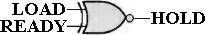My drawing above uses an XNOR gate. However, I wasn't sure if it satisfies the original question because the output of an XNOR gate can be low for two conditions per the truth table below.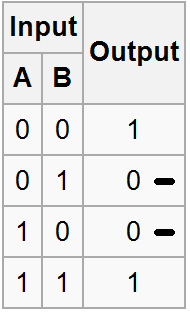Is there any way to design a logic circuit to satisfy the top input being *only* high and the bottom input being *only* low? In other words, I need the XNOR functionality excluding the second row where A=0, B=1, and Output=0.

Homework Helper
Gold Member
No that would be wrong because of the reason you give.

Are you allowed to you use more than one gate? It can be done with two NAND gates for example.

Gold Member
There is nothing in the textbook that says I must use only one gate.

Gold Member
It can be done with two NAND gates for example.

How would I chain two NANDs together to get the correct result?

Could I take the NAND output from the LOAD and READY and then push that output into an inverter?

Like this: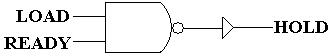Homework Helper
Gold Member
How would I chain two NANDs together to get the correct result?

Could I take the NAND output from the LOAD and READY and then push that output into an inverter?

Like this:Inverting a NAND gives you an AND gate. You tell us, would that work? Are you just guessing? Have you studied Karnaugh maps yet?

Gold Member
I have studied Karnaugh maps. That is the chapter I'm on. You're right about the NAND + inverter equaling an AND gate. I see that now.

I know how to plug create a Karnaugh map and get a minimized expresison, but I don't know what to do with that expression. I'm not being lazy, LCKurtz. I'm just unsure of what to do in this problem and need some help. This whole topic is brand new to me.

Your response was a bit rude, but thanks for pointing me in the direction of the Karnaugh maps.

Homework Helper
Gold Member
Your response was a bit rude....

I'm done helping you.

Gold Member
If you want to go by intuition, then you may want to notice that there is an asymmetry between the two inputs, which means that your circuit must treat the two input differently if you use standard gates as these all have symmetric inputs.

Homework Helper
Gold Member
How would I chain two NANDs together to get the correct result?

A hint...

Nand gate truth table..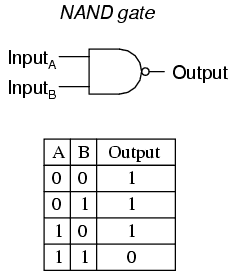What do you get if you connect both inputs to the READY signal?

Gold Member
A hint...

What do you get if you connect both inputs to the READY signal?

Connecting the READY signal to two NAND gate inputs yields a low. Also, this is the only case for a low in the AND gate (when the inputs are both high). Thanks CWatters... This is the push I needed.

I figured out the solution. It helped that I just finished a lab on combinational gates which I hadn't encountered prior to today.

My solution: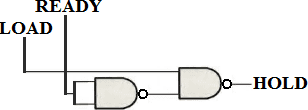The truth table for this diagram shows that a low is possible *only* when LOAD is HIGH and READY is LOW.

Homework Helper
Gold Member
Yes that's exactly the circuit I was hinting at.

Connecting the READY signal to two NAND gate inputs yields a low

Connecting two inputs together turns a NAND gate into an inverter. You could also achieve the same effect by connecting one of the inputs to Vcc = Logic 1. That would have the slight advantage that the load on the READY signal would be just one input rather than two.

Gold Member
You could also achieve the same effect by connecting one of the inputs to Vcc = Logic 1. That would have the slight advantage that the load on the READY signal would be just one input rather than two.

Very interesting... Thanks for the insight CWatters. I don't know why LCKurtz was so frustrated with me. I wouldn't want him as a teacher.

I see from the truth table what you're talking about in terms of using a NAND gate as an inverter. I'm assuming that building a circuit strictly out of NAND gates would be much cheaper than out of AND, OR, inverter, etc. gates.

Staff Emeritus